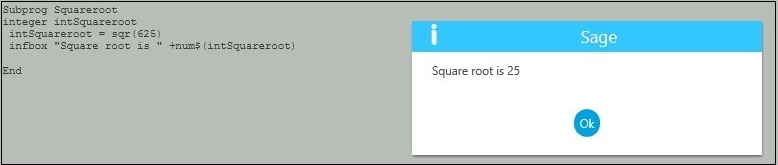# How to find the square root of the Number

By | February 15, 2017

The Sage X3 function holds the function which calculates the square root of the given number. Below is the syntax to calculate the same.

Syntax:

sqr(numeric value)

Example:The return type of function (i.e sqr()) is double if we will assign a value to integer data type it will parse the value to the integer. This numeric values can be used for further calculation.